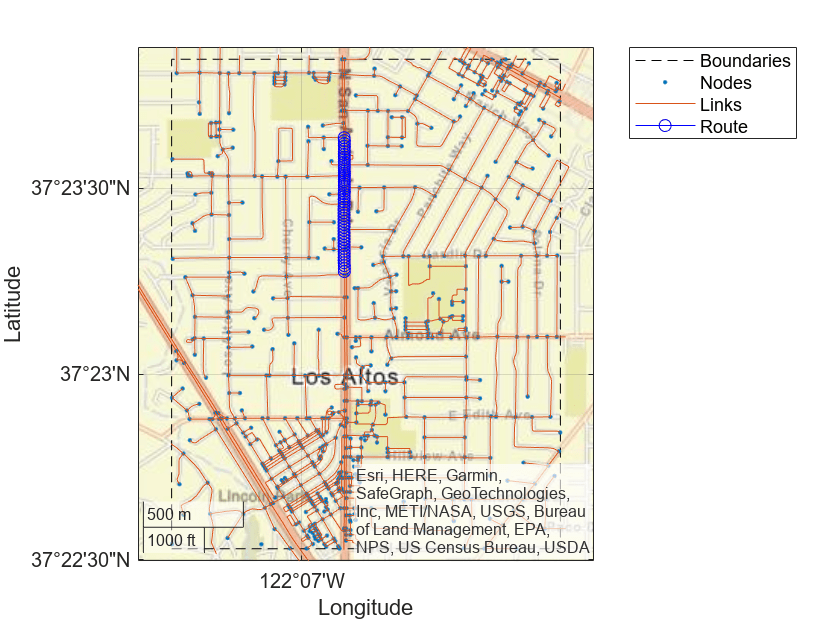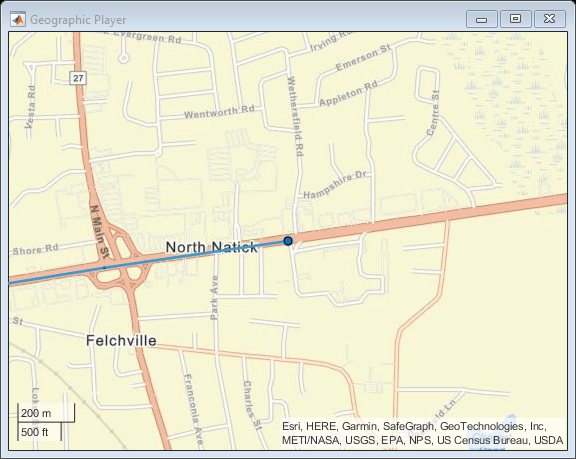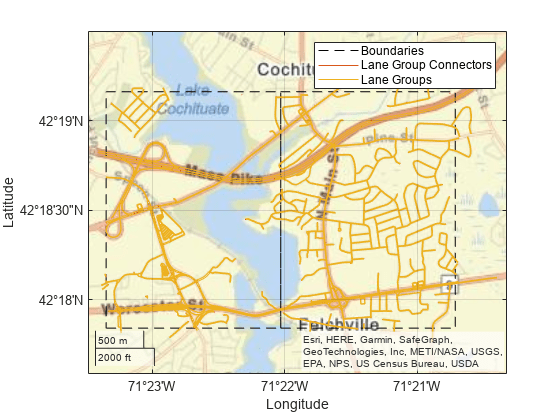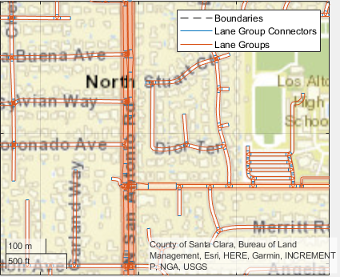# plot

Plot HERE HD Live Map layer data

## Syntax

``plot(layerData)``
``plot(layerData,'Axes',gxIn)``
``gxOut = plot(___)``

## Description

example

````plot(layerData)` plots HERE HD Live Map (HERE HDLM) layer data on a geographic axes. `layerData` is a map layer object that was read from the selected tiles of a `hereHDLMReader` object by using the `read` function.```

example

````plot(layerData,'Axes',gxIn)` plots the layer data in the specified geographic axes, `gxIn`.```

example

````gxOut = plot(___)` plots the layer data and returns the geographic axes on which the data was plotted, using the inputs from any of the preceding syntaxes. Use `gxOut` to modify properties of the geographic axes.```

## Examples

collapse all

Load a sequence of latitude and longitude coordinates from a driving route.

`data = load('geoSequence.mat')`
```data = struct with fields: latitude: [1000×1 double] longitude: [1000×1 double] ```

Create a HERE HD Live Map (HERE HDLM) reader from the specified coordinates. If you have not previously set up HERE HDLM credentials, a dialog box prompts you to enter them. The reader contains layered map data for the tile that the driving route is on.

`reader = hereHDLMReader(data.latitude,data.longitude);`

Read road topology data from the `TopologyGeometry` layer. Plot the data.

```roadTopology = read(reader,'TopologyGeometry'); plot(roadTopology) legend('Location','northeastoutside')```Overlay the driving route coordinates on the plot.

```hold on geoplot(data.latitude,data.longitude,'bo-','DisplayName','Route') hold off```Zoom in on the route.

```latcenter = median(data.latitude); loncenter = median(data.longitude); offset = 0.005; latlim = [latcenter-offset,latcenter+offset]; lonlim = [loncenter-offset,loncenter+offset]; geolimits(latlim,lonlim)```Use the HERE HD Live Map (HERE HDLM) service to read the lane topology data of a driving route and its surrounding area. Plot this data, and then stream the route on a geographic player.

Load the latitude and longitude coordinates of a driving route in Natick, Massachusetts, USA.

```route = load('geoSequenceNatickMA.mat'); lat = route.latitude; lon = route.longitude;```

Stream the coordinates on a geographic player.

```player = geoplayer(lat(1),lon(1),'HistoryDepth',5); plotRoute(player,lat,lon) for idx = 1:length(lat) plotPosition(player,lat(idx),lon(idx)) end```Create a HERE HDLM reader from the route coordinates. If you have not previously set up HERE HDLM credentials, a dialog box prompts you to enter them. The reader contains map data for the two map tiles that the route crosses.

`reader = hereHDLMReader(lat,lon);`

Read lane topology data from the `LaneTopology` layer of the map tiles. Plot the lane topology.

```laneTopology = read(reader,'LaneTopology'); plot(laneTopology)```Overlay the route data on the plot.

```hold on geoplot(lat,lon,'bo-','DisplayName','Route'); hold off```Overlay the lane topology data on the geographic player. Stream the route again.

```plot(laneTopology,'Axes',player.Axes) for idx = 1:length(lat) plotPosition(player,lat(idx),lon(idx)) end```Use the HERE HD Live Map (HERE HDLM) web service to read 3-D lane geometry data from a map tile. Then, plot the data on an OpenStreetMap® basemap.

Create a HERE HDLM reader for a map tile ID representing an area of Berlin, Germany. If you have not previously set up HERE HDLM credentials, a dialog box prompts you to enter them.

```tileID = uint32(377894435); reader = hereHDLMReader(tileID);```

Add the OpenStreetMap basemap to the list of basemaps available for use with the HERE HDLM service. After you add the basemap, you do not need to add it again in future sessions.

```name = 'openstreetmap'; url = 'https://a.tile.openstreetmap.org/\${z}/\${x}/\${y}.png'; copyright = char(uint8(169)); attribution = copyright + "OpenStreetMap contributors"; addCustomBasemap(name,url,'Attribution',attribution)```

Read 3-D lane geometry data from the `LaneGeometryPolyline` layer of the map tile. Plot the lane geometry on the `openstreetmap` basemap.

```laneGeometryPolyline = read(reader,'LaneGeometryPolyline'); gx = plot(laneGeometryPolyline); geobasemap(gx,'openstreetmap')```Zoom in on the central coordinate of the map tile.

```latcenter = laneGeometryPolyline.TileCenterHere3dCoordinate.Here2dCoordinate(1); loncenter = laneGeometryPolyline.TileCenterHere3dCoordinate.Here2dCoordinate(2); offset = 0.001; latlim = [latcenter-offset,latcenter+offset]; lonlim = [loncenter-offset,loncenter+offset]; geolimits(latlim,lonlim)```## Input Arguments

collapse all

HERE HDLM layer data to plot, specified as one of the layer objects shown in the table.

Layer ObjectDescriptionSample Plot
`LaneGeometryPolyline`

3-D lane geometry composed of a set of 3-D points joined into polylines.`LaneTopology`

Topologies of the HD Lane model, including lane group, lane group connector, lane, and lane connector topologies. This layer also contains the simplified 2-D boundary geometry of the lane model for determining map tile affinity and overflow.`TopologyGeometry`

Topology and 2-D line geometry of the road. This layer also contains definitions of the links (streets) and nodes (intersections and dead-ends) in the map tile.To obtain these layers from map tiles selected by a `hereHDLMReader` object, use the `read` function.

Geographic axes on which to plot data, specified as a `GeographicAxes` object.

## Output Arguments

collapse all

Geographic axes on which data is plotted, returned as a `GeographicAxes` object. Use this object to customize the map display. For more details, see GeographicAxes Properties.

#### Introduced in R2019a

 You need to enter into a separate agreement with HERE in order to gain access to the HDLM services and to get the required credentials (app_id and app_code) for using the HERE Service.